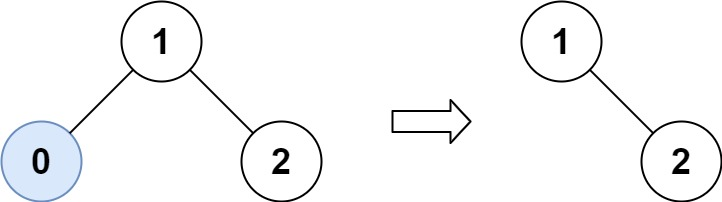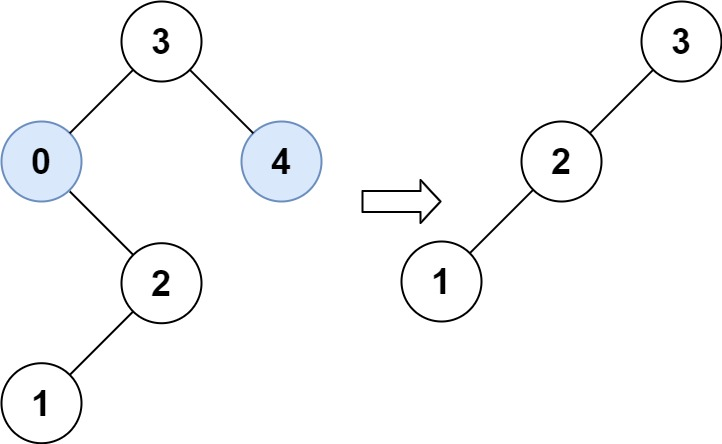# 669. Trim a Binary Search Tree

Trim a Binary Search Tree - LeetCode

Given the root of a binary search tree and the lowest and highest boundaries as low and high, trim the tree so that all its elements lies in [low, high]. Trimming the tree should not change the relative structure of the elements that will remain in the tree (i.e., any node’s descendant should remain a descendant). It can be proven that there is a unique answer. Return the root of the trimmed binary search tree. Note that the root may change depending on the given bounds.

Example 1:Input: root = [1,0,2], low = 1, high = 2 Output: [1,null,2] Example 2:Input: root = [3,0,4,null,2,null,null,1], low = 1, high = 3 Output: [3,2,null,1]

Constraints:

``````The number of nodes in the tree in the range [1, 104].
0 <= Node.val <= 104
The value of each node in the tree is unique.
root is guaranteed to be a valid binary search tree.
0 <= low <= high <= 104
``````

• code
``````class Solution:
def trimBST(self, root: Optional[TreeNode], low: int, high: int) -> Optional[TreeNode]:
def helper(root):
if not root: return
if low <= root.val <= high:
root.left = helper(root.left)
root.right = helper(root.right)
return root
elif root.val < low:
return helper(root.right)
elif root.val > high:
return helper(root.left)

return helper(root)
``````